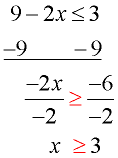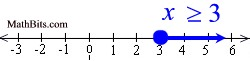Basic Linear Inequalities (single variable) MathBitsNotebook.com Terms of Use   Contact Person: Donna Roberts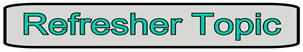You have already worked with inequality statements. Let's refresh those skills.

 Inequality Notations: (see other notation forms at Notations for Solutions) a > b ;    a is strictly greater than b a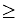b ;   a is greater than or equal to b a < b ;    a is striclty less than b a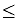b ;    a is less than or equal to b a ≠ b ;     a is not equal to b Hint: The "open" (larger) part of the inequality symbol always faces the larger quantity.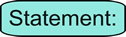If you can solve a linear equation, you can solve a linear inequality. The process is the same, with one exception ...

 ... when you multiply (or divide) an inequality by a negative value, you must change the direction of the inequality.

Let's see why this "exception" is actually needed.

 We know that 3 is less than 7. Now, lets multiply both sides by -1. Examine the results (the products). ... written 3 < 7. ... written (-1)(3) ? (-1)(7) ... written -3 ? -7 On a number line, -3 is to the right of -7, making -3 greater than -7.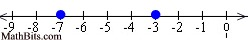-3 > -7 We have to reverse the direction of the inequality, when we multiply by a negative value, in order to maintain a "true" statement.When graphing a linear inequality on a number line, use an open circle for "less than" or "greater than", and a closed circle for "less than or equal to" or "greater than or equal to".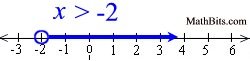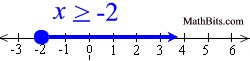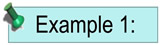Graph the solution set of:   -3 < x < 4 The solution set for this problem will be all values that satisfy both -3 < x and x < 4. Look for where the two inequalities overlap. Graph using open circles for -3 and 4 (since x can not equal -3 nor 4), and a bar to show the overlapping section.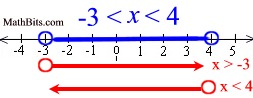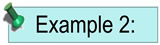Graph the solution set of:   x < -3 or x1 The solution set for this problem will be the full graph of both inequalities, since the two inequalities do not overlap. Notice that there is one open circle (for -3) and one closed circle (for 1).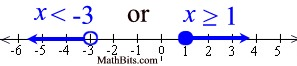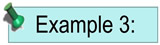Solve and graph the solution set of:   4x < 24 Proceed as you would when solving a linear equation: Divide both sides by 4. Note: The direction of the inequality stays the same since we did NOT divide by a negative value. Graph using an open circle for 6 (since x can not equal 6) and an arrow to the left (since our symbol is less than).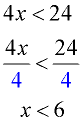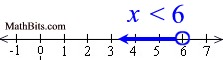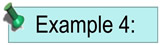Solve and graph the solution set of:   -5x25 Divide both sides by -5. Note: The direction of the inequality was reversed since we divided by a negative value. Graph using a closed circle for -5 (since x can equal -5) and an arrow to the left (since our final symbol is less than or equal to).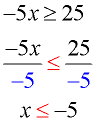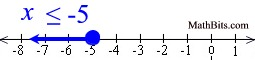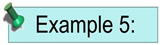Solve and graph the solution set of:   3x + 4 > 13 Proceed as you would when solving a linear equation: Subtract 4 from both sides. Divide both sides by 3. Note: The direction of the inequality stays the same since we did NOT divide by a negative value. Graph using an open circle for 3 (since x can not equal 3) and an arrow to the right (since our symbol is greater than).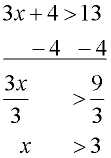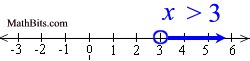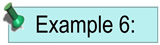Solve and graph the solution set of:   9 - 2x3 Subtract 9 from both sides. Divide both sides by -2. Note: The direction of the inequality was reversed since we divided by a negative value. Graph using a closed circle for 3 (since x can equal 3) and an arrow to the right (since our symbol is greater than or equal to).